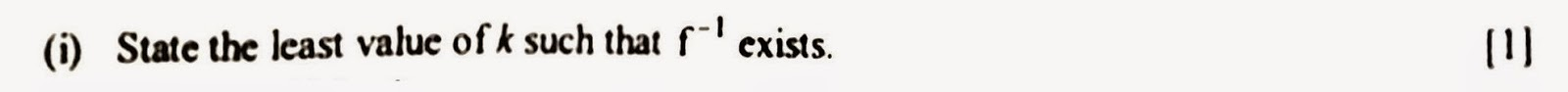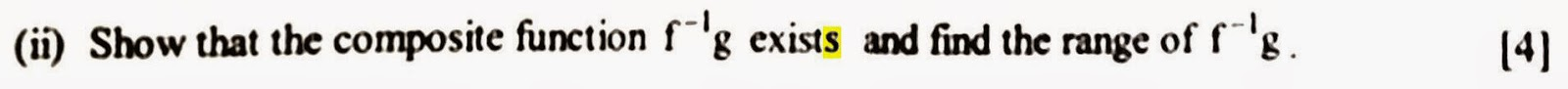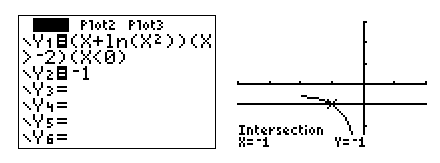## Sunday, March 29, 2015

### [AJC Promo 2012 Q5] Range of Composite Functions with InverseDiagram 1.  Whole Question

This is a question taken from Anderson Junior College, one of Singapore’s above-average junior colleges (in terms of the calibre of student intake).  By now this college is reputed to set the most difficult examination questions in Singapore.  It seems that they are trying to give the top junior colleges a run for their money, so to speak.  The question is difficult because it really tests students’ understanding of the concepts.  If you do not understand what is happening, you would be totally lost – even your graphing calculator (GC), the student’s favorite psychological crutch, would not be of much help.Diagram 2.  Part (i) of Question

Part (i) of the question tests students’ understanding of 1-to-1 functions (a.k.a. one-one functions or injective functions).  A function is invertible if and only if the function is one-one.  [ For the current A level H2 syllabus, it is assumed that the codomain is always the same as the range, so there is no need to worry about survjectivity. ]  Using the GC to graph the function  f   and to obtain the local maximum point, one sees that the required domain is  -2 < x < 0.
Ans:  k = -2.   [ I am using black for explanations and blue for written answers. ]
The domain is highlighted in yellow in the diagram below.  If the yellow region were to extend to the left beyond this point, it would be possible for a horizontal line to cross two points in the yellow region.  That would make the function  f  not 1-to-1 and hence not invertible.Diagram 3.  Graph of fDiagram 4.  Part (ii) of Question

For part (ii), we recall that a composite function exists if and only if the range of the first function (read from right to left) is a subset of the domain of the second function.
We require
 Range of g  Í  Domain of f-1
The domain of  f-1  (the second function) is actually the range of  f.  From the above diagram, the relevant part of  f(x)  goes from -¥  to  f(-2) = -2 + ln 4 = 2 ln 2 – 2 » -0.614.  The range of  g is everything from -1 downwards (see diagram below: imagine taking every possible point of  R, the domain of g on the x-axis and shooting them over to the y-axis).  We write
Range of g     = (-¥, -1]
Domain of f-1 = (-
¥, 2 ln 2 – 2]
Since Range of g Í Domain of f-1,  therefore  f-1g  exists.Diagram 5.  Range of g

Finding the range of composite functions is something that many students have difficulty with.  There are two methods: the direct method and the two-step method.  The direct method is usually difficult or infeasible.  In this case, finding range from the graph of  y = f-1g(x) is practically impossible, because there is no simple formula for  f-1.
 The two-step method: Step 1.  Find the range of the first function. Step 2.  Transfer this range to the x-axis of the graph of the second              function and map every point therein over to the y-axis.

Step 1 has been done already.  We have found that  Range of g = (-¥, -1] .Diagram 6.  The Two-step Method

For step 2, although the formula for  f-1  is impossible to find (it’s a pretty nasty question, isn’t it?), we know that this graph is a reflection of the graph of  y = f(x)  (shown in blue on the diagram on the right) in the line  y = x, and we can sketch this (shown in red on the diagram on the right).  Now transfer the range of g from the y-axis of your first diagram over to the x-axis of this diagram on the right (shown in green).  Now imagine taking every possible point of this set and mapping it over to the y-axis of the second graph.  The problem is: how to find  f-1(-1)  when we don’t even know the formula for f-1?  (really evil problem, isn’t it?).  One way to deal with this is to make an educated guess for  f(what?) = -1.
Notice that f(-1) = -1.  Therefore  f-1(-1) = -1.
What if your intuition really sucks and you cannot make a guess?  The GC can come to your rescue.  Set up the graph of  y = f(x)  with the restricted domain and then intersect that with the graph of  y = -1.  The intesection is at  x = -1,  which means f(-1) = -1,  or  f-1(-1) = -1,  as above.Diagram 7.  Using Intersection on the GC

Going back to diagram 6: From the range of g on the x-axis of the graph on the right, the points will land on every point from 0 down to f-1(-1) = -1, including -1 but excluding 0 (because x-axis is an asymptote for  y = f-1(x)).  We answer thus:-
Range of  f-1g = [-1, 0)Diagram 8.  Part (iii) of Question

Part (iii) tests students understanding of increasing and decreasing functions.
 f  is an increasing function means      whenever   a > b,   f(a) > f(b)   f  is an decreasing function means      whenever   a > b,   f(a) < f(b)
These are in fact the definitions of increasing and decreasing functions.  One can recognise an increasing function from its graph by the up slope (positive gradients) as you move from left to right.  For a decreasing function, the slope will be down as you move from left to right (negative gradients).  [An interesting note:  if  f  is a decreasing function,  then  f-1  will also be a decreasing function.]
In our case,  the graph of  f  is down-sloping, so it is a decreasing function.
Since  f  is a decreasing function,  whenever   a > b,   f(a) < f(b).
Once again we seem to have the pernicious problem of not knowing formula for  f-1.  How to solve the inequality then?  Well, we can apply  f  to both sides of the inequality and the inequality reverses (because f is a decreasing function).  Note that  f  and  f-1  “cancel”  as functions  i.e.  ff-1(w) = w  for whatever the  w  is as long as it is well-defined.  Hence we proceed as follows
f-1g(x) > -1
ff-1g(x) < f(-1)
g(x) < -1
-1 - x2 < -1
- x2 < 0
This latter inequality is the last trick on the question-setter sleeve desgined to unsettle the student.  How do you solve this inequality?  Do you need to equate or intersect with anything?  Anyway, what is the meaning of solve?
 To solve an inequality means to find all the possible values of  x  such that when you substitute each value into the inequality, the inequality becomes a true statement.
Note here that if we substituted  x = 0,  we would get  0 < 0, which is not true.  However, if we substituted any other real number,  x2  would always be a positive number, the LHS would always be a negative number, which is less than zero.  Conclusion:  x  can be any real number except 0.
Ans:  x Î R \ {0}

Note that the written solution (the parts typed in blue) is actually very short, although the explanation is rather long, because a lot of deep thinking is involved.

Reflection
Let us think back on the lessons learnt while solving this particularly difficult problem.
*  the reason why this problem seems difficult is because it tests students’ understanding of
concepts (which most are weak in).  From experience with many cohorts of students, the
JC teachers know what concepts students are weak in and they like to set questions that
exploit the chinks in students armours.
*  remember the horizontal line test and domain restriction to get a 1-to-1 function, so that the
function is invertible.
*  remember the condition for the existence of composite functions
*  inverse functions swap the domain and range with the original functions
*  the two-step method is recommended for finding range of composite functions
*  increasing functions preserve inequalities, while decreasing functions reverse
inequalities.  You can recognise a decreasing function from the downward
slope of its graph as you go from left to right.
Although you do not have the formula for  f-1,
*  the value of  f-1(-1)  can be found by intersecting the graph of  y = f(x)  with the horizontal line
y = -1.
*  f  and  f-1  “cancel” each other.
Finally,
*  what is the meaning of “solve an inequality”?
*  How to solve inequalities like  -x2 < 0?  What about  -x2 > 0?   x2 > 0?